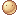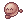# What is 2 and 2 put togeher?

97 views
asked Oct 2, 2019 in Other
I would like to see how many views this gets for this question.
commented Oct 3, 2019 by SnickerDoodles
PANT,PANT! I DON"T KNOW!!!!!!!!!!!!!!!!!!!!!!!!!!!!!!!!!!!!!!!!!!
commented Oct 3, 2019 by username

4 i think

commented Oct 4, 2019 by SnickerDoodles
nah, it's not 4. it's 222222222222222222222222222222222222222222222222222222222222222222222222222222222222222222222222222222222222222222222222222222222222222222222222222222222222222222222222222222222222222222222222222222222222222222222222222222222222222

answered Oct 10, 2019 by someone
selected Oct 10, 2019

Well... if x = 2 and x= pi and pi= 3.14159.., then it is 2+2 = x+x= Pi+Pi= 3.14159... + 3.14159... but 22 = 4, but u never know if there is a secret 2 hiding behind the plus so, 2+2+2= 6, 222= 484, 222= 8,388,608, and that’s three equations so triangle has three pointy bits and the angle of a triangle is 60* so you need to go:

8388608 % 484= 17,331.83471074380165, and then 60%6= 10 so we go, 10x17,331.83471074380165= 173,318.34710743801653. But we round to the 3 d.p which is 173,318.347. Now we subtract 4. 173,314.347. Then I count up how many ‘4’ I can make w. 173,314.347 cos 4 is a very special number in this certain equation. There are 4 ‘4’s but if I add 7+7=14 so there is 5 ‘4’s and then 1+3=4 so 6 ‘4’s and 6 ‘4’s equal 24 and 24 x Pi is the same as 24 x 3.14159 so it equals not ‘Pi Pi Pi Pi’ but 75.39822368615504. But that number is too big for ur maths teacher so to make him/her/unidentifiedalien happy you round to 2 d.p. So you get 75.39 and carry the one=

75.4!

Me smrt.

commented Oct 10, 2019 by (88,240 points)
0.0 that's a lotta stuff for 2 and 2 put together. Wow. Good job.
commented Oct 10, 2019 by (227,070 points)
OMG!! GREAT JOB!
commented Oct 10, 2019 by someone
me smrt
commented Nov 6, 2019 by unicorn girl

Me:Her:commented Feb 3 by (11,630 points)
Ummmmm... first, that was a lot. Second, "Me smrt"

is very informal. And that is not how you spell smart.
answered Oct 3, 2019 by (420,080 points)
You’ll probably get quite a bit
answered Oct 3, 2019 by (326,030 points)
I think it's 22?
answered Oct 3, 2019 by (55,410 points)
Huh? 2 plus 2? UMM..........
answered Oct 9, 2019 by (64,910 points)
The answer is fish, y’all. try it. turn the two’s on their sides